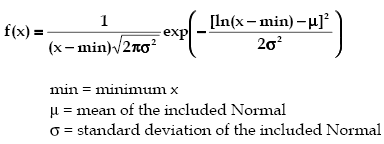AnyLogic

# lognormalThe Lognormal distribution is a continuous distribution bounded on the lower side. It is always 0 at minimum x, rising to a peak that depends on both mu and sigma, then decreasing monotonically for increasing x.

By definition, the natural logarithm of a Lognormal random variable is a Normal random variable. Its parameters are usually given in terms of this included Normal.

The Lognormal distribution can also be used to approximate the Normal distribution, for small sigma, while maintaining its strictly positive values of x [actually (x-min)].

The Lognormal distribution is used in many different areas including the distribution of particle size in naturally occurring aggregates, dust concentration in industrial atmospheres, the distribution of minerals present in low, concentrations, duration of sickness absence, physicians’ consultant time, lifetime distributions in reliability, distribution of income, employee retention, and many applications modeling weight, height, etc.(see Johnson et. al.1)

### lognormal(double mu, double sigma, double min)

Description
Generates a sample of the Lognormal distribution.
Parameters
Name Type of value Description
mu double The mean of the included Normal.
sigma double The standard deviation of the included Normal.
min double The minimum x value.
Result
Type Description
double The generated sample.

### lognormal(double mu, double sigma, double min, java.util.Random r)

Description
Generates a sample of the Lognormal distribution using the specified random number generator.
Parameters
Name Type of value Description
mu double The mean of the included Normal.
sigma double The standard deviation of the included Normal.
min double The minimum x value.
r java.util.Random The random number generator.
Result
Type Description
double The generated sample.

This document includes content from the “Stat::Fit User’s Manual”. Copyright 2016 Geer Mountain Software Corp.